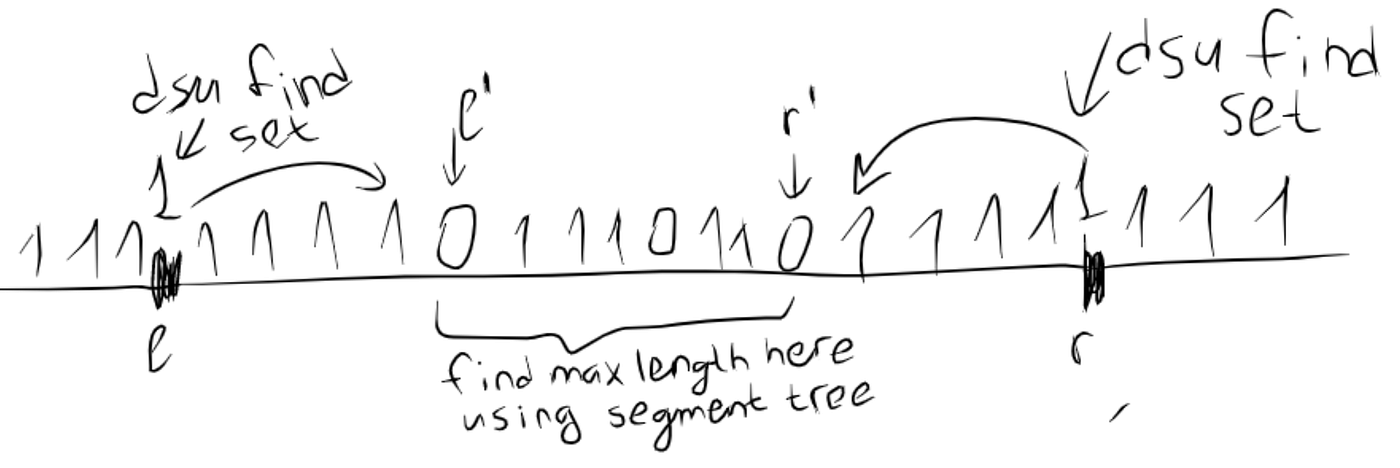Today was an upsolving day. There were some tasks that had been implemented before, but hadn’t got AC.

One of these tasks is B from Umnik’s Day 3. The idea of solution came from his analysis. The problem is: you have an array h of length n and m queries of type (l, r, w) — maximal minimum among all segments of length w inside range [l, r]. 1 ≤ w ≤ r — l + 1.

Solution: let’s do parallel binary search, that is binary search by answer for each query simultaneously. Binary search query is element X and l, r. We should answer maximal length of segments inside range [l, r] that consist of elements ≥ X. We put 1 if element X ≥ 1, 0 — else. So we want maximal segment of ones inside [l, r]. So we will do scanline by X in descending order. For some new X we put 1 in all positions of X in array h. Then if we have query X, l, r, we should do calculations like in the picture below:how to answer query (X, l, r), when all elements ≥ X is equal to 1

To be able to join segments and answer what segment consists of this point we need disjoint set union (DSU) and to find maximal length of segments strictly inside some range we need segment tree (ST) on maximum. So do scanline log(ans) times and maintain binary search queries for each problem’s query. Everything is with help of DSU and ST.

My solutions had been getting WAs because of the bug. My realization of joining point in DSU was so, that I didn’t any changes if this point is not connected to some existing segment. That’s absolutely wrong, because I need to put this point in DSU and update ST with 1 in this point.

Conclusion: EVERY TIME THINK ABOUT CORNER CASES!!!

Task C from Umnik’s Day 3. String of digits and sequence of operations are given. Every operation is digit->string. You should say the number, represented by resulting string by modulo. I saw no way how to do it moving from operation i to operation i + 1. But what if we will iterate from the last operation to the first? Then you can maintain for each digit in what string it turns into and the size of this string. That’s all we needed. Great.

Conclusion: SOMETIMES YOU SHOULD PROCESS SOMETHING FROM THE END INSTEAD OF BEGINNING